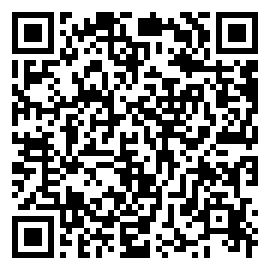Jimmy Zhang Apr 08, 2017
•• 端点处取值有意义且不为0
• 端点处取值无意义且区域无穷
• 端点处的取值为0 【最常见的情况】

P.S. 如果你有《高考数学你真的掌握了吗》系列丛书的《函数》一册，不妨先看看书再来看本文。

1. (《高考数学你真的掌握了吗 - 函数》 例5.18）若$f(x) = ax^2 - (3 - a)x + 2 - a > 0$$[0,1]$上恒成立，则实数$a$的取值范围是？

$\begin{cases}$
f(0) = 2 - a > 0 \
f(1) = a - 1 > 0
\end{cases}

$1 < a < 2$

$\Delta < 0 \Rightarrow 1 < a < \frac{9}{5}$

$\begin{cases}$
f(0) = 2 - a \ge 0 \
f(1) = a - 1 \ge 0
\end{cases}

$\lim\limits_{\Delta x \to 0} \frac{\Delta y}{\Delta x} = \lim\limits_{\Delta x \to 0} \frac{f(x_0 + \Delta x) - f(x_0)}{\Delta x}$

$f’(x_0) = \lim\limits{\Delta x \to 0} \frac{\Delta y}{\Delta x} = \lim\limits_{\Delta x \to 0} \frac{f(x_0 + \Delta x) - f(x_0)}{\Delta x}$

• 张杨文主编.高考数学你真的掌握了吗 函数 [M].北京：清华大学出版社, 2014 (2016.5 重印)

This blog is under a CC BY-NC-SA 4.0 Unported License.